# How to Calculate and Solve for Rate | Salt Trace Method | Water Budget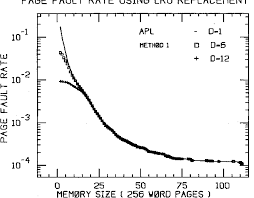The image above represents rate.

To compute for rate, three essential parameters are needed and these parameters are Rate of injection for salt solution (q), Concentration of salt solution (c1) and Concentration of river downstream (c2).

The formula for calculating rate:

Q = q(c1 – c2)

Where:

Q = Rate
q = Rate of Injection for Salt Solution or Trace
c1 = Concentration of Salt Solution or Trace
c2 = Concentration of River Downstream

Let’s solve an example;
Find the rate when the rate of injection for salt solution is 12, the concentration of salt solution is 20 and the concentration of river downstream is 18.

This implies that;

q = Rate of Injection for Salt Solution or Trace = 12
c1 = Concentration of Salt Solution or Trace = 20
c2 = Concentration of River Downstream = 18

Q = q(c1 – c2)
Q = 12(20 – 18)
Q = 12(2)
Q = 24

Therefore, the rate is 24.

Calculating the Rate of Injection for Salt Solution when the Rate, the Concentration of Salt Solution and the Concentration of River Downstream is Given.

q = Q / (c1 – c2)

Where;

q = Rate of Injection for Salt Solution or Trace
Q = Rate
c1 = Concentration of Salt Solution or Trace
c2 = Concentration of River Downstream

Let’s solve an example;
Find the rate of injection for salt solution when the rate is 40, the concentration of salt solution is 32 and the concentration of river downstream is 14.

This implies that;

Q = Rate = 40
c1 = Concentration of Salt Solution or Trace = 32
c2 = Concentration of River Downstream = 14

q = Q / (c1 – c2)
q = 40 / (32 – 14)
q = 40 / 18
q = 2.2

Therefore, the rate of injection for salt solution is 2.2.

Calculating the Concentration of Salt Solution when the Rate, the Rate of Injection for Salt Solution and the Concentration of River Downstream is Given.

c1 = (Q / q) + c2

Where;

c1 = Concentration of Salt Solution or Trace
Q = Rate
q = Rate of Injection for Salt Solution or Trace
c2 = Concentration of River Downstream

Let’s solve an example;
Given that the rate is 52, the rate of injection for salt solution is 22 and the concentration of river downstream is 10. Find the concentration of salt solution?

This implies that;

Q = Rate = 52
q = Rate of Injection for Salt Solution or Trace = 22
c2 = Concentration of River Downstream = 10

c1 = (Q / q) + c2
c1 = (52 / 22) + 10
c1 = 2.36 + 10
c1 = 12.36

Therefore, the concentration of salt solution is 12.36.

Calculating the Concentration of River Downstream when the Rate, the Rate of Injection for Salt Solution and the Concentration of Salt Solution is Given.

c2 = c1 – (Q / q)

Where;

c2 = Concentration of River Downstream
Q = Rate
q = Rate of Injection for Salt Solution or Trace
c1 = Concentration of Salt Solution or Trace

Let’s solve an example;
Find the concentration of river downstream when the rate is 24, the rate of injection for salt solution is 12 and the concentration of salt solution is 8.

This implies that;

Q = Rate =24
q = Rate of Injection for Salt Solution or Trace = 12
c1 = Concentration of Salt Solution or Trace = 8

c2 = c1 – (Q / q)
c2 = 8 – (24 / 12)
c2 = 8 – 2
c2 = 6

Therefore, the concentration of river downstream is 6.

Nickzom Calculator – The Calculator Encyclopedia is capable of calculating the rate | salt trace method.

To get the answer and workings of the rate | salt trace method using the Nickzom Calculator – The Calculator Encyclopedia. First, you need to obtain the app.

You can get this app via any of these means:

To get access to the professional version via web, you need to register and subscribe for NGN 1,500 per annum to have utter access to all functionalities.
You can also try the demo version via https://www.nickzom.org/calculator

Apple (Paid) – https://itunes.apple.com/us/app/nickzom-calculator/id1331162702?mt=8
Once, you have obtained the calculator encyclopedia app, proceed to the Calculator Map, then click on Agricultural under Engineering.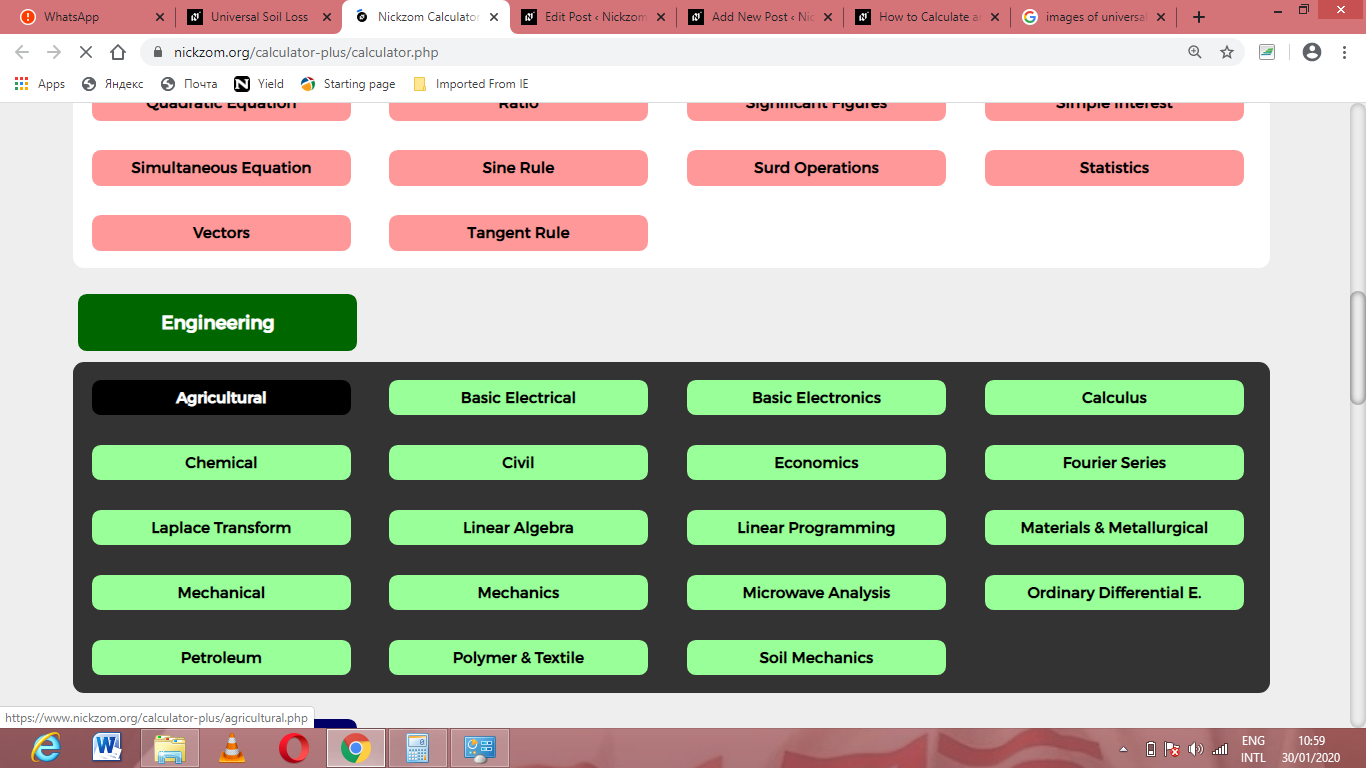Now, Click on Water Budget under Agricultural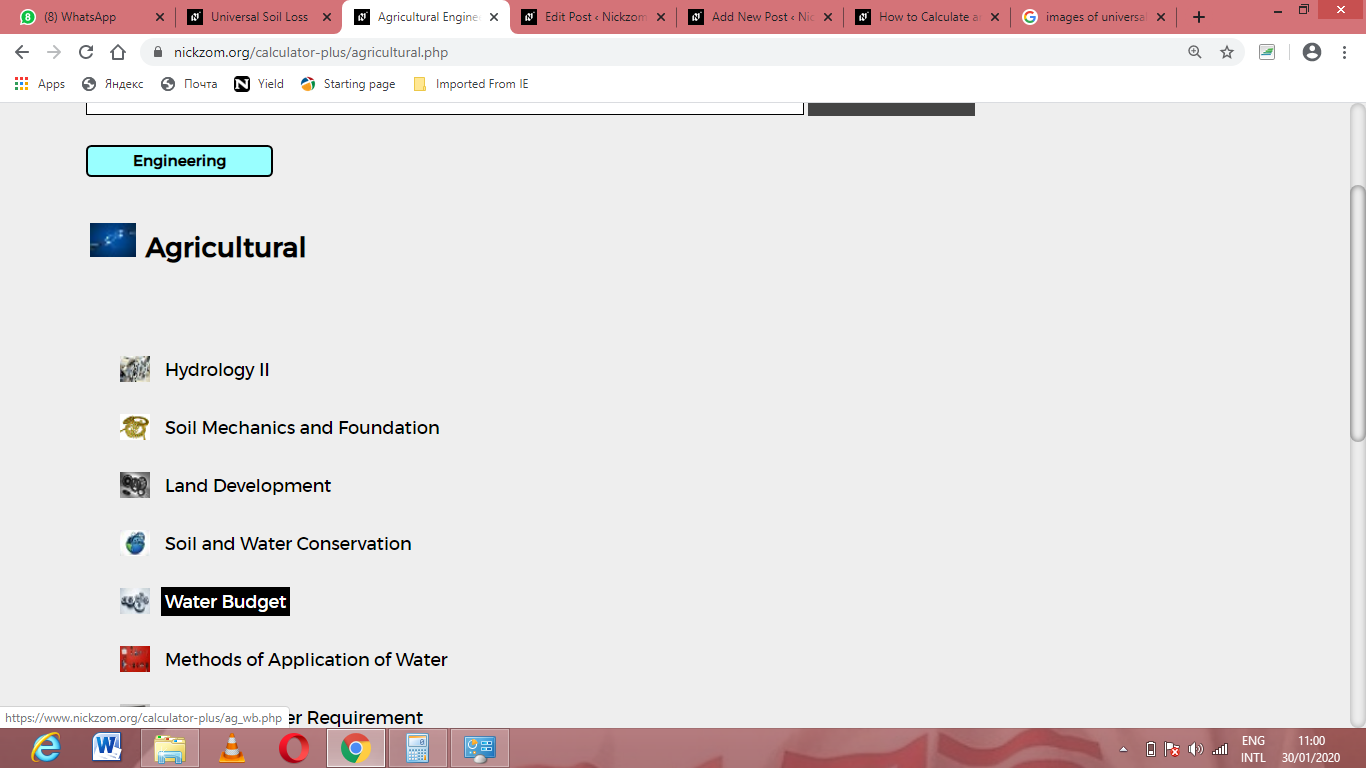Now, Click on Rate| Salt Trace Method under Water Budget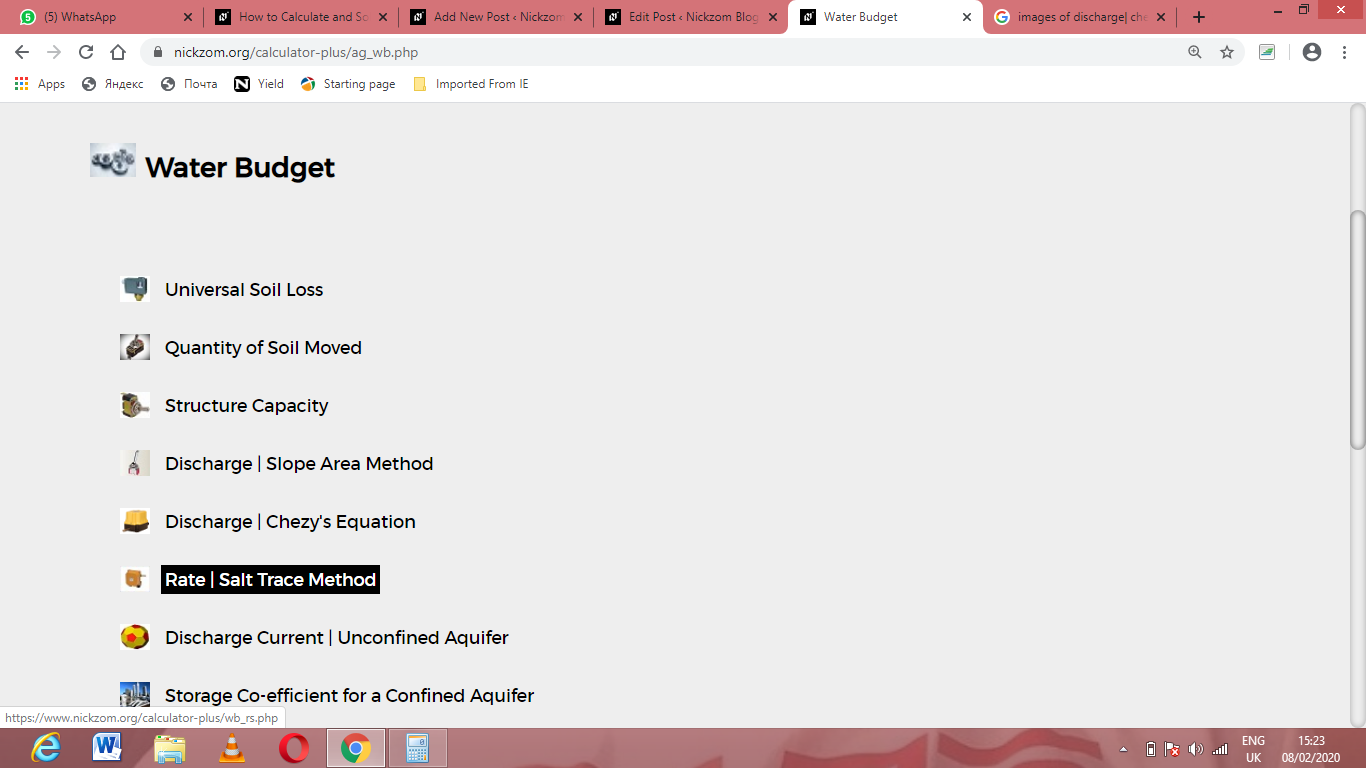The screenshot below displays the page or activity to enter your values, to get the answer for the rate | salt trace method according to the respective parameters which are the Rate of injection for salt solution (q), Concentration of salt solution (c1) and Concentration of river downstream (c2).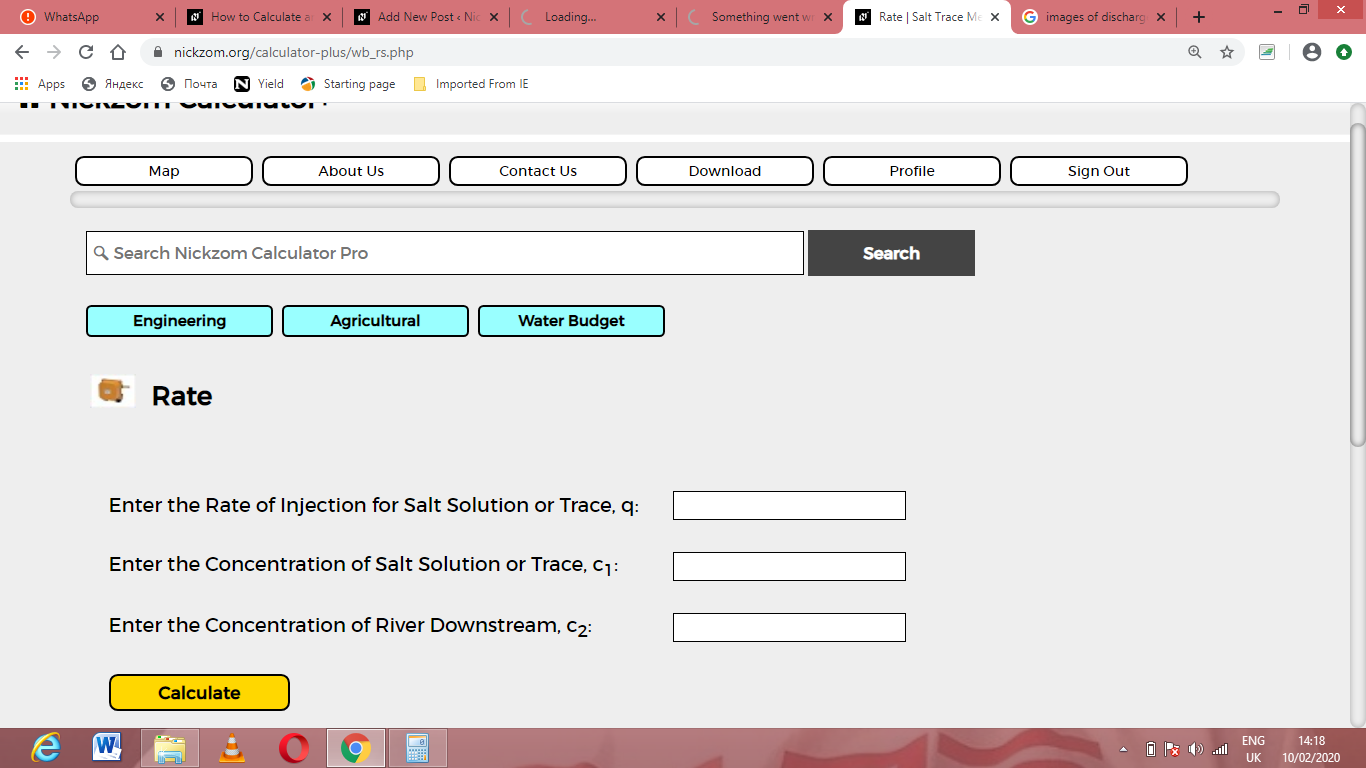Now, enter the values appropriately and accordingly for the parameters as required by the Rate of injection for salt solution (q) is 12, Concentration of salt solution (c1) is 20 and Concentration of river downstream (c2) is 18.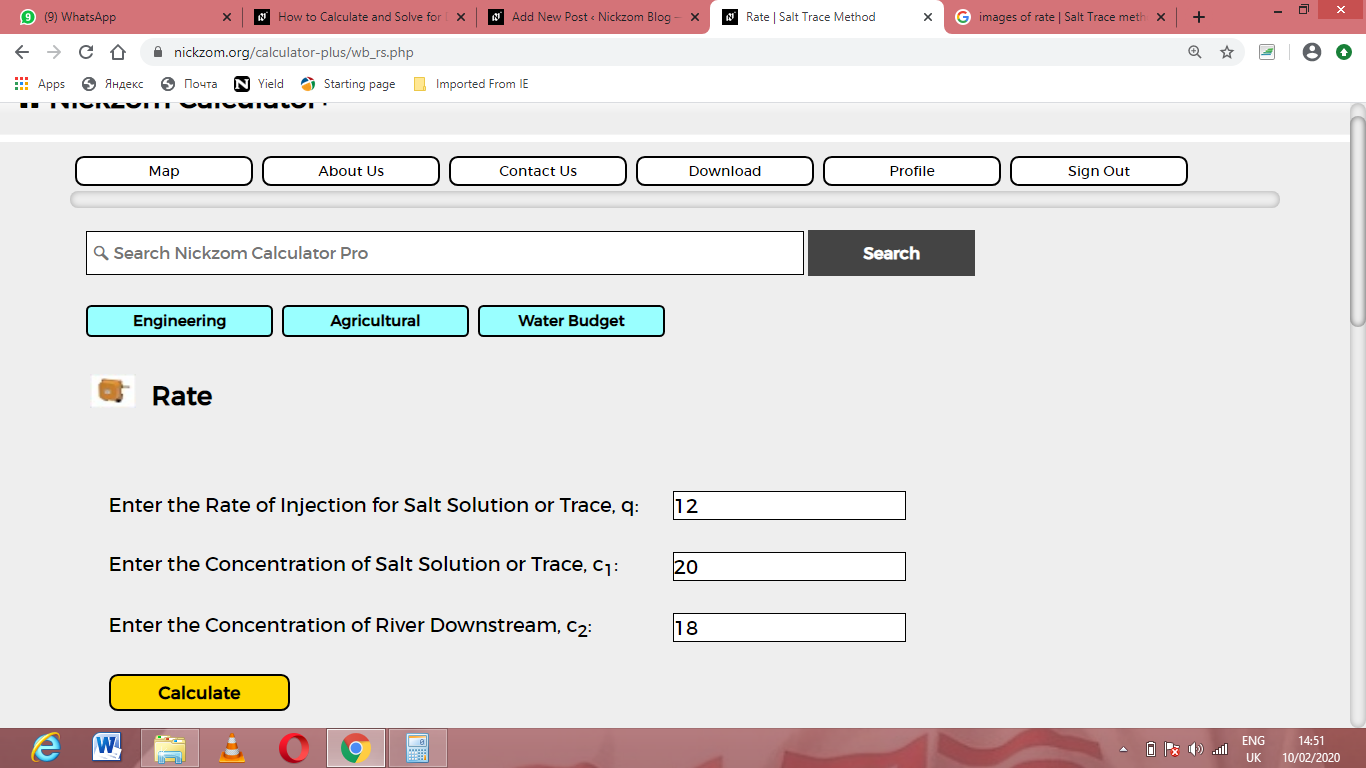Finally, Click on Calculate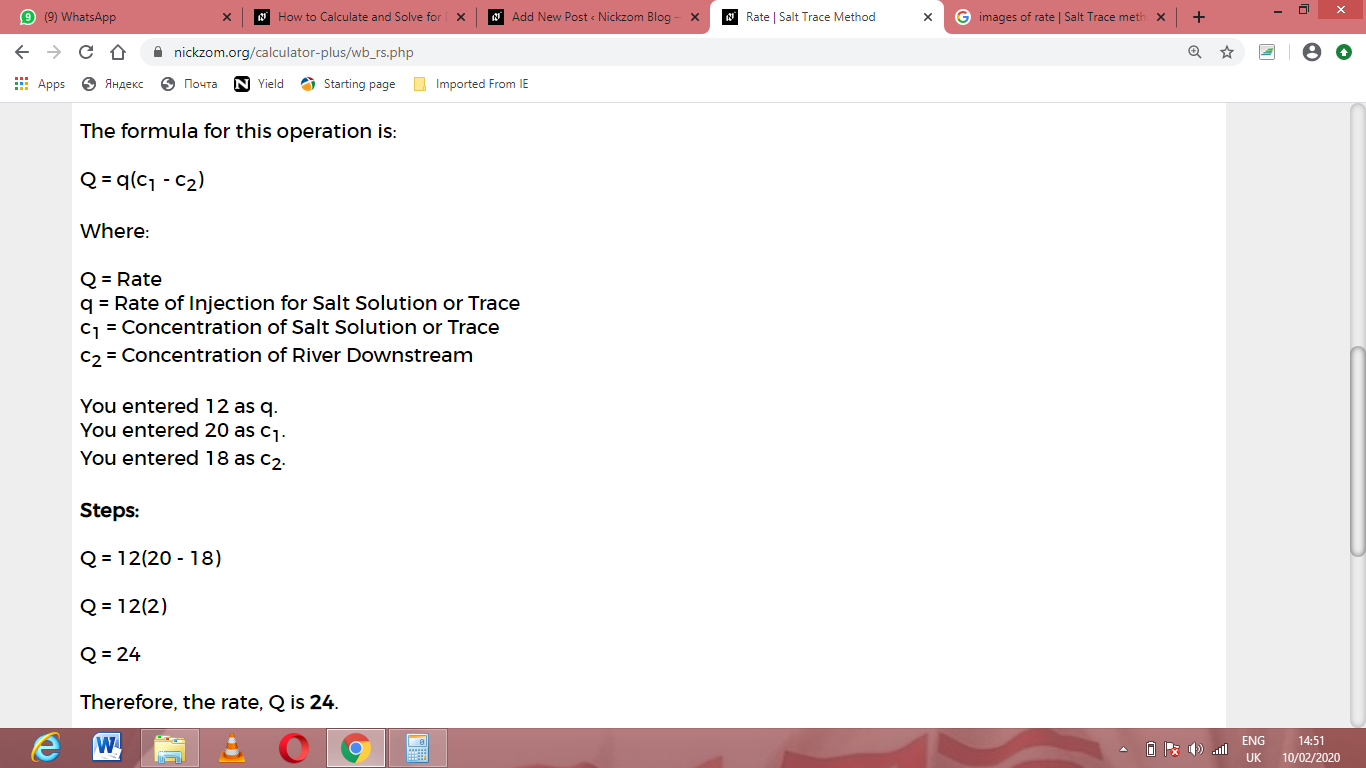As you can see from the screenshot above, Nickzom Calculator– The Calculator Encyclopedia solves for the rate | salt trace method and presents the formula, workings and steps too.